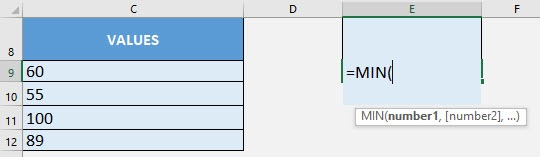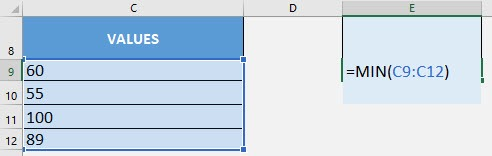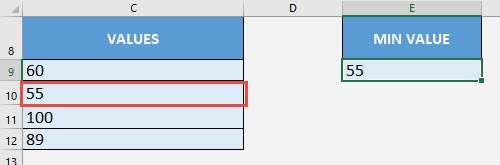What does it do?

Get the smallest value from a range of values

Formula breakdown:

=MIN(number1, [number2], …)

What it means:

=MIN(a number or range of values, [additional numbers], …)

If you want to get the smallest value out of a list of values, just one use of Excel’s MIN Formula gives you the answer instantly!

I explain how you can do this below:STEP 1: We need to enter the MIN function in a blank cell:

## =MIN(STEP 2: The MIN arguments:

## number1, [number2], …

Where is the list of values?

Select the cells containing the values that you want to get the minimum value from.

## =MIN(C9:C12)You now have the minimum value of 55 from the list!How to Use the MIN Formula in Excel